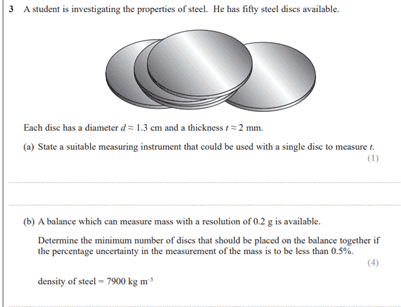# Ocr practical paper question on concept

• bonbon22

#### bonbon22

Homework Statement
problem statemetn below
Relevant Equations
volume = surface area of sphere * thickness
percentage uncertainty = absolute uncertainty/ valueshould n't it be a maximum number to achieve less than 0.5% uncertainty for the last question as you add more disks would there not be more uncertanity therefore as the number of disks for 0.5% is 9.5, 10 would give an unceranity of greater than 0.5% so it should be 9? the answer is 10.

What's an ocr? Optical character recognition?

What's an ocr? Optical character recognition?
Exam broad,, for a levels . IF you got free time friend would be grateful if you could help me out.

should n't it be a maximum number to achieve less than 0.5% uncertainty for the last question as you add more disks would there not be more uncertanity therefore as the number of disks for 0.5% is 9.5, 10 would give an unceranity of greater than 0.5% so it should be 9? the answer is 10.
An implicit assumption going in is that the discs are identical.

The uncertainty is from the limited resolution of the scale: 0.2 grams. As specified, the scale's resolution is independent of how many discs you pile on. The more you pile on, the lower the uncertainty per disk.

•berkeman and bonbon22